Fractal Art Gallery Magnification Series I Home Art Math Business

Here is a series of twelve julia set fractals with their magnifications below them.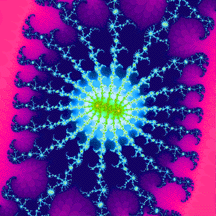1 1,900 105,625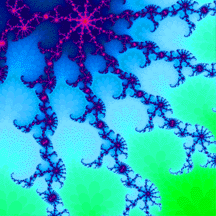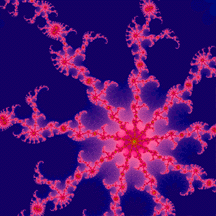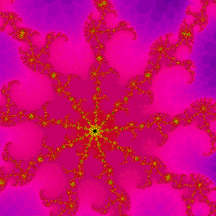6,760,000 300 million 7.5 billion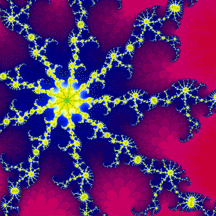270 billion 6.76 trillion 169 trillion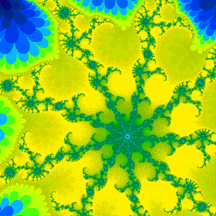16.9 quadrillion 1.69 quintillion 169 quintillion

All of the images here are Julia sets of the equation f(z) = z^2 + c,
where c = .3577911 + .0629241i.

This series exhibits the principle of self-similarity, that is, the deeper you go into a fractal,
the more it remains the same.

As a note, a quintillion has eighteen zeroes! (169,000,000,000,000,000,000)